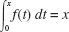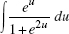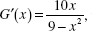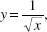# AP Calculus BC Practice Test 32

### Test Information9 questions18 minutes

Calculator Disallowed

1. As the tides change, the water level in a bay varies sinusoidally. At high tide todayat 8 A.M., the water level was 15 feet; at low tide, 6 hours later at 2 P.M., it was 3 feet. How fast, in feet per hour, was the water level dropping at noontoday?

2. Letsin πx. Then f (3) =

3.is equal to

4. Given f (x) = log10x and log10(102)2.0086, which is closest to f (100)?

5. If G(2) = 5 andthen an estimate of G(2.2) using a tangent-line approximation is

6. The area bounded by the parabola y = x2 and the lines y = 1 and y = 9 equals

7. The first-quadrant region bounded byy = 0, x = q (0 < q < 1), and x = 1 is rotated about the x-axis. The volume obtained as q →0+ equals

8. A curve is given parametrically by the equations

x = 3 - 2sint and y = 2cos t - 1.

The length of the arc from t = 0 to t = π is

9. Suppose the graph of f is both increasing and concave up on axb. Then, using the same number of subdivisions, and with L, R, M, and T denoting, respectively, left, right, midpoint, and trapezoid sums, it follows that